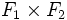# External direct product of formal group laws

Suppose$R$ is a commutative unital ring. Suppose$F_1$ and$F_2$ are formal group laws over$R$ of dimensions$d_1$ and$d_2$ respectively. The external direct product of$F_1$ and$F_2$, which we denote$F_1 \times F_2$, is a formal group law of dimension$d_1 + d_2$, given by:$(F_1 \times F_2)(x_1,x_2,\dots,x_{d_1},x_{d_1 + 1},\dots,x_{d_1+d_2},y_1,y_2,\dots,y_{d_1},y_{d_1+1},\dots,y_{d_1+d_2})$$= (F_1(x_1,x_2,\dots,x_{d_1},y_1,y_2,\dots,y_{d_1}),F_2(x_{d_1 + 1},\dots,x_{d_1+d_2},y_{d_1+1},\dots,y_{d_1+d_2}) )$
In other words, the first$d_1$ coordinates of$F_1 \times F_2$ are obtained by applying$F_1$ to the first$d_1$ coordinates of the two inputs, and the last$d_2$ coordinates of$F_1 \times F_2$ are obtained by applying$F_2$ to the last$d_2$ coordinates of the two inputs.